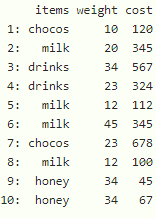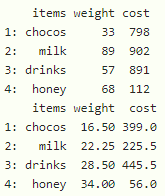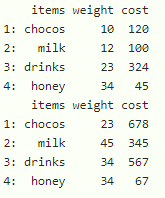# Summarize Multiple Columns of data.table by Group in R

• Last Updated : 23 Sep, 2021

In this article, we will discuss how to summarize multiple columns of data.table by Group in R Programming Language.

## R

 `# load data.table package``library``(``"data.table"``)`` ` `# create data table with 3 columns``# items``# weight and #cost``data <- ``data.table``( items= ``c``(``"chocos"``,``"milk"``,``"drinks"``,``"drinks"``,``                             ``"milk"``,``"milk"``,``"chocos"``,``"milk"``,``                             ``"honey"``,``"honey"``),    ``                    ` `                   ``weight= ``c``(10,20,34,23,12,45,23,``                             ``12,34,34),``                    ` `                   ``cost=  ``c``(120,345,567,324,112,345,``                            ``678,100,45,67))`` ` `# display``data`

Output:## We can summarize the multiple columns in 4 ways:

• By finding average
• By finding sum
• By finding the minimum value
• By finding the maximum value

we can do this by using lapply() function

Syntax: datatable[, lapply(.SD, summarizing_function), by = column]

where

• datatable is the input data table
• lpply() is used to hold two parameters
• first parameter is .SD is standard R object
• second parameter is an summarizing function that takes summarizing functions to summarize the datatable
• by is the name of the column in which data is grouped based on this column

## R

 `# load data.table package``library``(``"data.table"``)`` ` `# create data table with 3 columns``# items``# weight and #cost``data <- ``data.table``( items= ``c``(``"chocos"``,``"milk"``,``"drinks"``,``"drinks"``,``                             ``"milk"``,``"milk"``,``"chocos"``,``"milk"``,``                             ``"honey"``,``"honey"``),    ``                    ` `                   ``weight= ``c``(10,20,34,23,12,45,23,``                             ``12,34,34),``                    ` `                   ``cost=  ``c``(120,345,567,324,112,345,``                            ``678,100,45,67))`` ` ` ` `# group by sum with items column``print``(data[, ``lapply``(.SD, sum), by = items])`` ` `# group by average with items column``print``(data[, ``lapply``(.SD, mean), by = items])`

Output:## R

 `# load data.table package``library``(``"data.table"``)`` ` `# create data table with 3 columns``# items weight and #cost``data <- ``data.table``( items= ``c``(``"chocos"``,``"milk"``,``"drinks"``,``"drinks"``,``                             ``"milk"``,``"milk"``,``"chocos"``,``"milk"``,``                             ``"honey"``,``"honey"``),    ``                    ` `                   ``weight= ``c``(10,20,34,23,12,45,23,``                             ``12,34,34),``                    ` `                   ``cost=  ``c``(120,345,567,324,112,345,``                            ``678,100,45,67))`` ` `# group by minimum  with items column``print``(data[, ``lapply``(.SD, min), by = items])`` ` `# group by maximum with items column``print``(data[, ``lapply``(.SD, max), by = items])`

Output:My Personal Notes arrow_drop_up